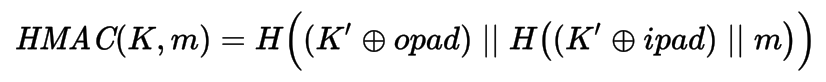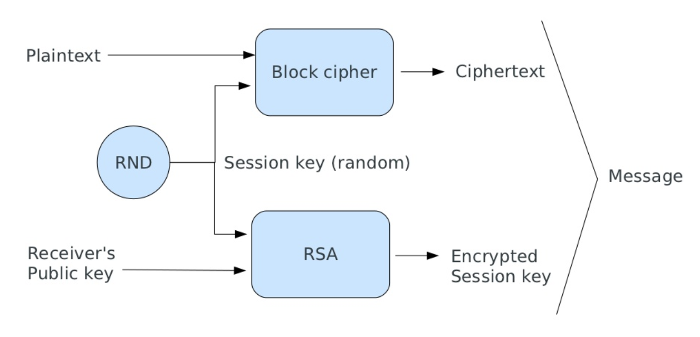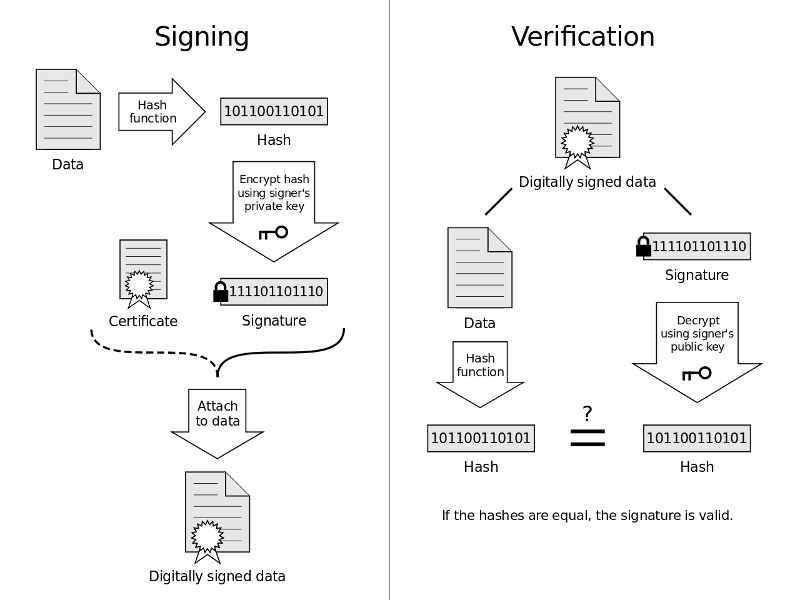## Encryption, authentication and data integrity in PHP 7

by Enrico Zimuel
Senior Software Engineer
Rogue Wave Software, Inc.

phpCE 2017, Rawa Mazowiecka (Poland), Nov 3Web developer since 1996 Senior Software Engineer at Rogue Wave Software Inc. Core team of Apigility, Expressive and Zend Framework TEDx and international speaker Research Programmer at Amsterdam University Co-founder of PUG Torino (Italy)

## Cryptography is hard!

• Use only standard algorithms (e.g. AES)
• Use well known libraries (e.g. OpenSSL, libsodium)
• Be updated, attacks are around the corner

## Cryptography in PHP

• Mcrypt, deprecated since PHP 7.1
• OpenSSL extension, public key and symmetric encryption
• Sodium extension, from PHP 7.2+

## OpenSSL

• Ciphers: RSA, AES, CAMELLIA, DES, RC2, RC4, check with openssl_get_cipher_methods()
• Block modes: CBC, CFB, CTR, ECB, XTS
• Authenticated encryption: GCM, CCM (PHP 7.1+)

## Sodium

• Authenticated encryption
• Stream encryption/file encryption
• Public-key cryptography
• Key derivation/Key exchange

## Authenticated Encryption

• Encryption only provides confidentiality
• An attacker can easily alter the encrypted message
• Padding Oracle Attacks can be applied to retrieve the plaintext without the key!

## Authenticated Encryption

PHP 7.1 provides AEAD support for OpenSSL: Galois/Counter Mode (GCM), Counter CBC-MAC (CCM)

``````
openssl_encrypt (\$data, \$method, \$key, \$options = 0, \$iv = "",
&\$tag = null, \$aad = "", \$tag_len = 16)

openssl_decrypt (\$data, \$method, \$key, \$options = 0, \$iv = "",
\$tag = null, \$aad = "")
``````

## Example: encrypt

``````
function encrypt(string \$data, string \$key): string
{
\$iv = random_bytes(16); // size for aes-256-gcm
\$ciphertext = openssl_encrypt(
\$data,
'aes-256-gcm',
\$key,
OPENSSL_RAW_DATA,
\$iv,
\$tag
);
if (false === \$ciphertext) {
throw new Exception ('Error on encrypt');
}
return \$iv . \$tag . \$ciphertext;
}
``````

## Example: decrypt

``````
function decrypt(string \$ciphertext, string \$key): string
{
\$plaintext = openssl_decrypt(
mb_substr(\$ciphertext, 32, null, '8bit'),
'aes-256-gcm',
\$key,
OPENSSL_RAW_DATA,
mb_substr(\$ciphertext, 0, 16, '8bit'),
mb_substr(\$ciphertext, 16, 16, '8bit')
);
if (false === \$plaintext) {
throw new Exception('Error on decrypt');
}
return \$plaintext;
}
``````

## Encryption keys

• If possible use random bytes, e.g. random_bytes()
• PBKDF2 (Password-Based Key Derivation Function 2) is a key derivation function RFC 2898
• hash_pbkdf2(\$algo, \$password, \$salt, \$iterations, \$length=0, \$raw=false)

• Suggestion: use SHA-256 as \$algo
• In 2017 a reasonable \$iterations is around 80k

## HMAC

• Hash-based message authentication code (RFC 2140)where H is hash function, K is key, K' derivated key, opad is outer padding (0x5c), ipad is inner padding (0x36), ⊕ is XOR, and || is concatenation
• In PHP:
``````
hash_hmac (\$algo, \$data, \$key, \$raw_output = false)
hash_hmac_file (\$algo, \$filename, \$key, \$raw_output = false)
``````
• Suggestion: use SHA-256 as \$algo

## Hybrid cryptosystem

• Public key algorithms are too slow to encrypt a full text message
• We need a different approach (Hybrid cryptosystem):
• generate a random session key;
• encrypt the session key using a public key algorithm;
• encrypt the message with a symmetric cipher using the session key;
• send the ciphertext and the encrypted session key;## Public and private key

``````
// Generate public and private keys
\$keys = openssl_pkey_new(array(
"private_key_bits" => 4096,
"private_key_type" => OPENSSL_KEYTYPE_RSA,
));

// Store the private key in a file
\$passphrase = 'test';
openssl_pkey_export_to_file(\$keys, 'private.key', \$passphrase);

// Store the public key in a file
\$details   = openssl_pkey_get_details(\$keys);
\$publicKey = \$details['key'];
file_put_contents('public.key', \$publicKey);
``````

## Hybrid encryption

``````
\$msg = 'This is the secret message';
// Encryption
\$key = random_bytes(32);
openssl_public_encrypt(\$key, \$encKey, \$publicKey);
\$ciphertext = encrypt(\$msg, \$key);
file_put_contents('encrypted.msg', \$encKey . \$ciphertext);
// Decryption
\$ciphertext = file_get_contents('encrypted.msg');
\$encKey     = mb_substr(\$ciphertext, 0, 512, '8bit');
\$ciphertext = mb_substr(\$ciphertext, 512, null, '8bit');
\$privateKey = openssl_pkey_get_private(
'file:///path/to/private.key',
\$passphrase // to access the private key
);
openssl_private_decrypt(\$encKey, \$key, \$privateKey);
\$result = decrypt(\$ciphertext, \$key); // \$result === \$msg
``````

## Digital signature

• How to provide authentication and non-repudiation?
• A digital signature is a mathematical scheme for demonstrating the authenticity of a digital message or document
• Commonly used for software distribution, financial transactions, and in other cases where it is important to detect forgery or tampering## Signature with OpenSSL

``````
// Compute the signature
\$priKey = openssl_pkey_get_private(
'file:///path/to/private.key',
\$passphrase
);
\$data = file_get_contents('/path/to/file_to_sign');
openssl_sign(\$data, \$sign, \$priKey, "sha256");
printf("Signature : %s\n", base64_encode(\$sign));

// Verify the signature
\$pubKey = openssl_pkey_get_public(
'file:///path/to/public.key'
);
\$result = openssl_verify(\$data, \$sign, \$pubKey, "sha256");

echo \$result === 1 ? 'Signature ok' : 'Signature not valid';
``````

• Never use a simple hash (e.g. MD5, SHA1), even + salt
• Use bcrypt or Argon2
• The default PASSWORD_DEFAULT is bcrypt, at the moment

## Thanks!

Contact me: enrico [at] zend.com